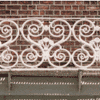#### You may also like### Frieze Patterns in Cast Iron

A gallery of beautiful photos of cast ironwork friezes in Australia with a mathematical discussion of the classification of frieze patterns.### The Frieze Tree

Patterns that repeat in a line are strangely interesting. How many types are there and how do you tell one type from another?### Friezes

Some local pupils lost a geometric opportunity recently as they surveyed the cars in the car park. Did you know that car tyres, and the wheels that they on, are a rich source of geometry?

# Reflecting Lines

### Why do this problem?

This problem helps students to consolidate their understanding of how equations of the form $y=mx+c$ describe the gradient and position of lines. Students explore the effect on the equation of reflecting a straight line. Students are encouraged to visualise the movement of the graphs in order to conjecture and test their conjectures.

This is good preparation for future work on transforming the graphs of more complicated functions.

### Possible approach

It is useful if students have done some preliminary work plotting straight line graphs. The problems Parallel Lines and Diamond Collector offer good starting points. This problem works well alongside Translating Lines.

Working with the whole group demonstrate the interactivity. Decide on the position of one of the lines. Ask the class to describe how to create the reflection of the line in each of the axes. How can they be sure they have reflected correctly?

Give students plenty of time, perhaps working in pairs at computers, to explore the effect that reflections have on the equations of lines. Clarify to the students that ultimately, the challenge is to be able to predict the new equation whenever a straight line is reflected in one of the axes.

Later, bring the class together and use the interactivity to test their ability to do this. Do this a number of times with different lines, and reflections in either axis, until students are able to predict the new equation with confidence. Ask them to share insights and explanations/justifications.

Hand out this card matching activity to check that students are confident in pairing reflected graphs and identifying the line of symmetry. If students need more practice they can create their own sets of cards for their peers to pair off.

Introduce the final part of the problem: can students predict the equation of the resulting line when an original line is reflected in one axis and the image is then reflected in the other axis? Expect them to explain and justify their findings, perhaps by producing a poster for display or making a presentation to the class.

### Key questions

When we reflect a graph, what changes? What stays the same?

How does this affect the equation of the graph?
What is the effect of two combined reflections?

### Possible support

Ensure that students are secure about the relationship between a line's properties and its equation. Encourage students to sketch the graphs of different equations and then use the interactivity to test their predictions.

### Possible extension

Choose a straight line that doesn't go through the origin. Reflect in one of the axes. Reflect both lines in the other axis.

What shape is enclosed by the four lines? What is its area?
Find a way to predict the area from the equation of the first line, without drawing any graphs.

Students could now try the problem Surprising Transformations